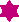# User Forum

Subject :IMO    Class : Class 9The angle between internal and the external bisectors of an angle is ______.

(A) 90°

(B) 180°

(C) 270°

(D) 30°

## Ans 1:

Class : Class 10
Let the angle be x. Ext angle of x=180-x ATQ, req angle=x/2+((180-x)/2)=180/2=90(ans) opt a

## Ans 2:

Class : Class 10
Let the angle be x. Ext angle of x=180-x ATQ, req angle=x/2+((180-x)/2)=180/2=90(ans) opt a